Home WindowsMS-Office Microsoft Excel : Text Functions Part -3

# Microsoft Excel : Text Functions Part -3

0 comment 554 views

Hi,

In previous post we have talked about some of the Text functions in detail. We will continue this post from where we left.

If you have not read previous posts, this post might be some confusing to you. I will request you to read these posts before proceeding.

Text Functions in Microsoft Excel Part -1

Text Functions in Microsoft Excel Part –2

Directly moving ahead to function details and their use.

#### 9. FIXED()

Introduction: FIXED function Rounds a number to the specified number of decimals, formats the number in decimal format using a period and commas, and returns the result as text.
Using Method: This function takes minimum of one and maximum of 3 parameters. First one is Number, this can be any number value or reference to a cell. Second is the decimals , for rounding. Last one is a Boolean option for keeping period separator or not.

=FIXED(139493.4532,2) or =FIXED(A1,-1,TRUE)

Example: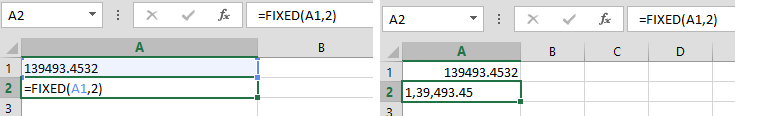NOTE: Difference between ROUND function and FIXED function is, FIXED converts number to text after rounding but ROUND keep number to number after rounding.

#### 10. LEFT()

Introduction: LEFT function returns specified number of characters from start based in start number (length) in a string.

Using Method: This function takes two parameters. First one is text to be searched, this can be simple string value or reference to a cell. Second is the number of characters to start.
=LEFT(“Hello World”,6) or =LEFT(A1,6)

Example: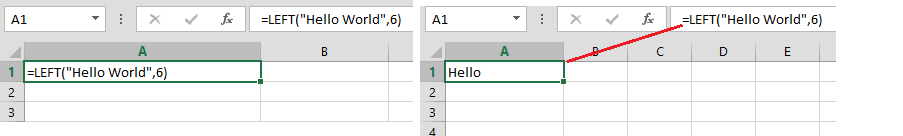#### 11. LEN()

Introduction: LEN function returns number of characters in a string. In simple words, it returns the length of a string.

Using Method: This function takes single parameter and that is of type string or reference to a cell.

=LEN(“Hello World”) or =LEN(A1)

Example: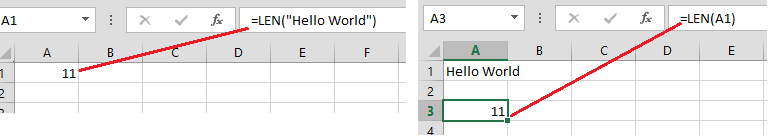#### 12. LOWER()

Introduction: LOWER function converts all characters in a string to lower case.
Using Method: This function takes a single parameter and that is of type string or reference to a cell.

=LOWER(“Hello World”) or =LOWER(A1)

Example: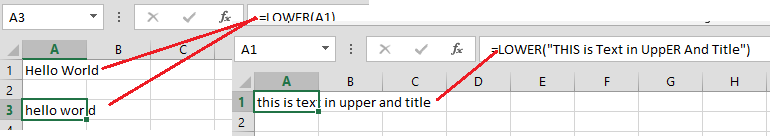#### 13. MID()

Introduction: MID function returns the middle characters from a string specified by start number and length.
Using Method: This function takes three parameters. One is text which is required, another is Start number to search from and another is number of character to show or length to be picked.
=MID(“John is from Nepal”,9,10) or =MID(A1,9,10)

Example: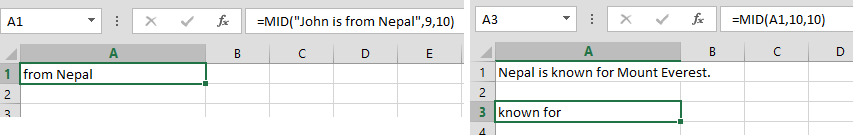#### 14. PROPER()

Introduction: PROPER function converts all characters and words to Title case as per English Grammar. Means all the beginning character of all words are in capital letter and other are in small letter regardless of their typography.
Using Method: This function takes a single parameter and that is text string or reference to cell .

=PROPER(“this is going to BE CHANGED in title”) or =PROPER(A1)

Example: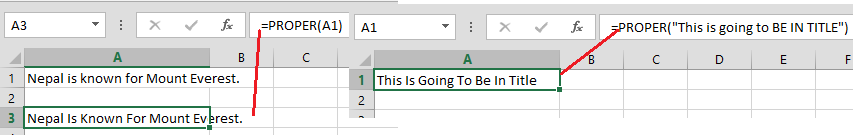#### 15. REPLACE()

Introduction: REPLACE function replaces a part of string with specified string.
Using Method: This function takes four parameters, First one is text to be replaced, then starting number of character where replace to be started, then number of character to replace (length), and final one is new text.
=REPLACE(A1,6,3,”was ”) or =REPLACE(A1,6,3,C1)

Example:#### 16. RIGHT()

Introduction: RIGHT function does the exactly same work as LEFT do but from opposite index. This returns the text from end of an string based on the length passed by user.
Using Method: This function takes two parameters and both are required. First one is string or reference to cell containing text and another is number of character to be shown.
=RIGHT(“The last five words”,5) or =RIGHT(A1,5)

Example: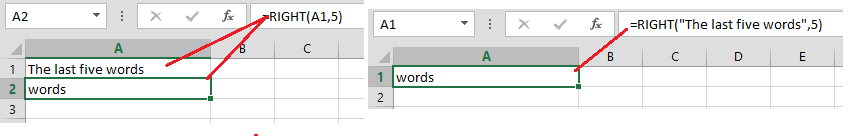I will be back with remaining text function in another post. Thanks for reading.

You can provide feedback using comments or contact page.

John Bhatt

0 comment